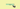# JavaScript — Get the Last element in an arrayIn this tutorial, we are going to learn about how to get the last element in an array using JavaScript.

Consider we have an array with 4 elements like this.

``const users = ['sai','jim','alex','era'];``

Now, we need to get the last element from a `users` array.

Normally, we can get the last element by using that element index.

``users //  this is last element in array.``

This not a correct way, because we always manually count the number of elements in an array to find the last element.so that I’m showing you two different solutions to get the last element without knowing you how many elements in an array.

## First way: Using array.length property

In array, we have a `length` property by using that we can find how many elements present in an array if we subtract `arr.length-1` we can get the last element.

Example:

``````const users = ['sai','jim','alex','era'];

const lastElement = users[arr.length-1];

console.log(lastElement); // 'era'``````

## Second way: Using es6 slice method

If we pass `-1` as an argument to the slice method we can get the last element in an array.

Example:

``````const users = ['sai','jim','alex','era'];
const lastElement = ...users.slice(-1);

console.log(lastElement); // 'era'``````